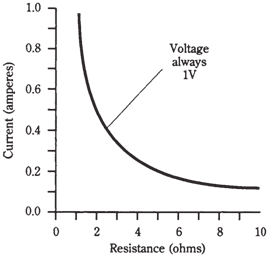## Resistance Assignment Help

Assignment Help: >> Electrical Engineering - Resistance

Resistance:

Resistance is the measure of the opposition which a circuit provides to the flow of electric current. You can compare it to diameter of a hose. Actually for metal wire, this is an perfect analogy: small-diameter wire has larger resistance, and large-diameter wire has less resistance. Certainly the type of metal makes a difference too. Iron wire has higher resistance for the given diameter than copper wire. Nichrome wire has more resistance still.

The standard unit of resistance is ohm. This is abbreviated by the upper-case  Greek letter omega, resembling the upside down capital U (Ω).

Electric wire is rated for resistivity sometimes. The standard unit for this purpose is the ohm per foot or the ohm per meter. You might also come across the unit ohm per kilometer.  Table given below shows the resistivity for various common sizes of wire.

When the 1V is placed across the 1 ohm of resistance, supposing that the power supply can

Table --Resistivity for copper wire, in terms of the size in American Wire Gauge (AWG).

 Wire size, AWG 2 Resistivity, ohms/km 0.52 4 0.83 6 1.3 8 2.7 10 3.3 12 5.3 14 8.4 16 13 18 21 20 34 22 54 24 86 26 140 28 220 30 350

deliver infinite number of charge carriers, there will be current produced of 1A. If the resistance is made double, the current is cut in half. If the resistance is cut in half, the current doubles. Thus, the current flow, for a constant voltage, is inversely proportional to the resistance.  Figure given below is a graph that shows various currents, through various resistances, given a constant voltage of 1V across whole resistance.Figure--   Current versus resistance through an electric device when voltage is constant at 1 V.

Resistance has various properties in an electric circuit. If there is a current flowing through the resistive material, there will always be a potential difference across the resistive object. This is represented in Figure. The larger the current through resistor, the greater EMF across resistor. Generally EMF is proportional to the current through resistor. This behavior of resistors is very useful in the design of electronic  circuits.Figure--   Whenever a resistance carries a current, there is a voltage across it.

Electrical circuits have some resistance always. There is no such thing as a perfect or ideal conductor. When some metals are chilled to very low temperatures, they lose practically all of their resistance, but they never become perfect, resistance free conductors. This phenomenon, about which you may have heard, is generally known as superconductivity.  In recent years, some special metals have been found which behave this way even at moderate  temperatures.  Researchers  are trying to concoct substances which will superconduct even at the room temperature. Superconductivity is an active field in physics these days.

There is no such thing as a perfectly resistance-free substance, there is not a truly infinite resistance also. Even air conducts to some extent, although the effect is so small that it can be ignored. In some of the electronic  applications, materials are selected on the basis of how nearly infinite their resistance is. These materials can produce good electric insulators, and good dielectrics for capacitors, devices which store electric charge.

In electronics, the resistance of a component varies, depending on the conditions under which it can be operated. A transistor, for instance, might have high resistance some of the time, and low resistance at other times. This high/low fluctuation can be made to take place thousands/millions/billions of times each second. In this way, oscillators,  amplifiers and digital electronic devices function in radio receivers and transmitters, digital computers  telephone networks, and satellite links.